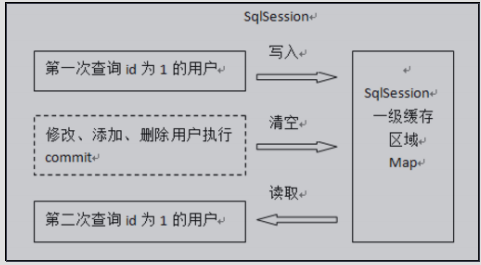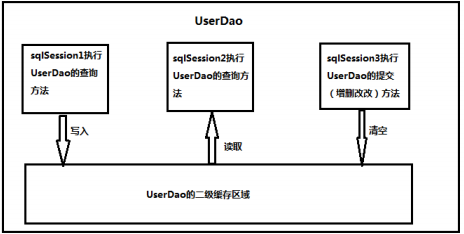# MyBatis 基础知识四分析

1.1 什么是延迟加载

``````延迟加载：就是在需要用到数据时才进行加载，不需要用到数据时就不加载数据。延迟加载也称懒加载.

1.2 延迟加载实现方式

1.2.1 使用 assocation 实现延迟加载

``````   <resultMap type="com.zzg.jdbc.mybatis.entity.Account"
id="accountExpand">
<id property="id" column="id" />
<result column="uid" property="uid" />
<result column="name" property="name" />
<result column="money" property="money" />
<!-- 它是用于指定从表方的引用实体属性的 -->
<association property="user" column="uid" select="selectByPrimaryKeyExpandUser"/>
</resultMap>

<select id="selectByPrimaryKeyExpand"  resultMap="accountExpand">
select a.* from account as a where a.id =1
</select>

select： 填写我们要调用的 select 映射的 id
column ： 填写我们要传递给 select 映射的参数``````

1.2.2 使用 Collection 实现延迟加载

``````<resultMap type="user" id="userMap">
<id column="id" property="id"></id>
<result column="username" property="username"/>
<result column="address" property="address"/>
<result column="sex" property="sex"/>
<result column="birthday" property="birthday"/>
<!-- collection 是用于建立一对多中集合属性的对应关系
ofType 用于指定集合元素的数据类型
select 是用于指定查询账户的唯一标识（账户的 dao 全限定类名加上方法名称）
column 是用于指定使用哪个字段的值作为条件查询
-->
<collection property="accounts" ofType="account"
select="com.itheima.dao.IAccountDao.findByUid" column="id">
</collection>
</resultMap>

<!-- 配置查询所有操作 -->
<select id="findAll" resultMap="userMap">
select * from user
</select>

<collection>标签：

select 属性：

column 属性：

Mybatis 中缓存分为一级缓存，二级缓存。2.1 一级缓存：一级缓存是 SqlSession 级别的缓存，只要 SqlSession 没有 flush 或 close，它就存在。

2.1.1 一级缓存分析2.2 二级缓存

SqlSession 可以共用二级缓存，二级缓存是跨 SqlSession 的。

2.2.1 二级缓存结构图``````首先开启 mybatis 的二级缓存。

sqlSession1 去查询用户信息，查询到用户信息会将查询数据存储到二级缓存中。

sqlSession2 去查询与 sqlSession1 相同的用户信息，首先会去缓存中找是否存在数据，如果存在直接从缓存中取出数据。``````

2.2.2 二级缓存开启流程

``````<settings>
<!-- 开启二级缓存的支持 --> <setting name="cacheEnabled" value="true"/>
</settings>

false 代表不开启二级缓存``````

``````<cache>标签表示当前这个 mapper 映射将使用二级缓存，区分的标准就看 mapper 的 namespace 值。
<?xml version="1.0" encoding="UTF-8"?>
<!DOCTYPE mapper
PUBLIC "-//mybatis.org//DTD Mapper 3.0//EN"
"http://mybatis.org/dtd/mybatis-3-mapper.dtd"> <mapper namespace="com.itheima.dao.IUserDao">
<!-- 开启二级缓存的支持 -->
<cache></cache>
</mapper>``````

``````<!-- 根据 id 查询 --> <select id="findById" resultType="user" parameterType="int" useCache="true">
select * from user where id = #{uid}
</select> 将 UserDao.xml 映射文件中的<select>标签中设置 useCache=”true”代表当前这个 statement 要使用

3.1 常用注解

``````@Insert:实现新增
@Update:实现更新
@Delete:实现删除
@Select:实现查询
@Result:实现结果集封装
@Results:[email protected]/* <![CDATA[ */!function(t,e,r,n,c,a,p){try{t=document.currentScript||function(){for(t=document.getElementsByTagName('script'),e=t.length;e--;)if(t[e].getAttribute('data-yjshash'))return t[e]}();if(t&&(c=t.previousSibling)){p=t.parentNode;if(a=c.getAttribute('data-yjsemail')){for(e='',r='0x'+a.substr(0,2)|0,n=2;a.length-n;n+=2)e+='%'+('0'+('0x'+a.substr(n,2)^r).toString(16)).slice(-2);p.replaceChild(document.createTextNode(decodeURIComponent(e)),c)}p.removeChild(t)}}catch(u){}}()/* ]]> */ 一起使用，封装多个结果集
@ResultMap:[email protected]/* <![CDATA[ */!function(t,e,r,n,c,a,p){try{t=document.currentScript||function(){for(t=document.getElementsByTagName('script'),e=t.length;e--;)if(t[e].getAttribute('data-yjshash'))return t[e]}();if(t&&(c=t.previousSibling)){p=t.parentNode;if(a=c.getAttribute('data-yjsemail')){for(e='',r='0x'+a.substr(0,2)|0,n=2;a.length-n;n+=2)e+='%'+('0'+('0x'+a.substr(n,2)^r).toString(16)).slice(-2);p.replaceChild(document.createTextNode(decodeURIComponent(e)),c)}p.removeChild(t)}}catch(u){}}()/* ]]> */ 定义的封装
@One:实现一对一结果集封装
@Many:实现一对多结果集封装
@SelectProvider: 实现动态 SQL 映射
@CacheNamespace:实现注解二级缓存的使用``````

3.2 基于注解实现增删改查

``````/**
*
* <p>Title: IUserDao</p>
* <p>Description: 用户的持久层接口</p>
* <p>Company: http://www.itheima.com/ </p>
*/
public interface IUserDao {
/**
* 查询所有用户
* @return
*/
@Select("select * from user")
@Results(id="userMap",
value= {
@Result(id=true,column="id",property="userId"),
@Result(column="username",property="userName"),
@Result(column="sex",property="userSex"),
@Result(column="address",property="userAddress"),
@Result(column="birthday",property="userBirthday")
})
List<User> findAll();
/*
* 根据 id 查询一个用户
* @param userId
* @return
*/
@Select("select * from user where id = #{uid} ")
@ResultMap("userMap")
User findById(Integer userId);
/**
* 保存操作
* @param user
* @return
*/
@Insert("insert into
user(username,sex,birthday,address)values(#{username},#{sex},#{birthday},#
{address})")
@SelectKey(keyColumn="id",keyProperty="id",resultType=Integer.class,before =
false, statement = { "select last_insert_id()" })
int saveUser(User user);

/**
* 更新操作
* @param user
* @return
*/
@Update("update user set
username=#{username},address=#{address},sex=#{sex},birthday=#{birthday} where id
=#{id} ")
int updateUser(User user);

/**
* 删除用户
* @param userId
* @return
*/
@Delete("delete from user where id = #{uid} ")
int deleteUser(Integer userId);

/**
* 查询使用聚合函数
* @return
*/
@Select("select count(*) from user ")
int findTotal();

/**
* 模糊查询
* @param name
* @return
*/
@Select("select * from user where username like #{username} ")
List<User> findByName(String name);
}

3.3 基于注解复杂关系映射

3.3.1 复杂关系注解说明：

``````@Results 注解

[email protected]/* <![CDATA[ */!function(t,e,r,n,c,a,p){try{t=document.currentScript||function(){for(t=document.getElementsByTagName('script'),e=t.length;e--;)if(t[e].getAttribute('data-yjshash'))return t[e]}();if(t&&(c=t.previousSibling)){p=t.parentNode;if(a=c.getAttribute('data-yjsemail')){for(e='',r='0x'+a.substr(0,2)|0,n=2;a.length-n;n+=2)e+='%'+('0'+('0x'+a.substr(n,2)^r).toString(16)).slice(-2);p.replaceChild(document.createTextNode(decodeURIComponent(e)),c)}p.removeChild(t)}}catch(u){}}()/* ]]> */ 注解，[email protected]/* <![CDATA[ */!function(t,e,r,n,c,a,p){try{t=document.currentScript||function(){for(t=document.getElementsByTagName('script'),e=t.length;e--;)if(t[e].getAttribute('data-yjshash'))return t[e]}();if(t&&(c=t.previousSibling)){p=t.parentNode;if(a=c.getAttribute('data-yjsemail')){for(e='',r='0x'+a.substr(0,2)|0,n=2;a.length-n;n+=2)e+='%'+('0'+('0x'+a.substr(n,2)^r).toString(16)).slice(-2);p.replaceChild(document.createTextNode(decodeURIComponent(e)),c)}p.removeChild(t)}}catch(u){}}()/* ]]> */ 集合
@Results（[email protected]/* <![CDATA[ */!function(t,e,r,n,c,a,p){try{t=document.currentScript||function(){for(t=document.getElementsByTagName('script'),e=t.length;e--;)if(t[e].getAttribute('data-yjshash'))return t[e]}();if(t&&(c=t.previousSibling)){p=t.parentNode;if(a=c.getAttribute('data-yjsemail')){for(e='',r='0x'+a.substr(0,2)|0,n=2;a.length-n;n+=2)e+='%'+('0'+('0x'+a.substr(n,2)^r).toString(16)).slice(-2);p.replaceChild(document.createTextNode(decodeURIComponent(e)),c)}p.removeChild(t)}}catch(u){}}()/* ]]> */（），@Result（）}）[email protected]/* <![CDATA[ */!function(t,e,r,n,c,a,p){try{t=document.currentScript||function(){for(t=document.getElementsByTagName('script'),e=t.length;e--;)if(t[e].getAttribute('data-yjshash'))return t[e]}();if(t&&(c=t.previousSibling)){p=t.parentNode;if(a=c.getAttribute('data-yjsemail')){for(e='',r='0x'+a.substr(0,2)|0,n=2;a.length-n;n+=2)e+='%'+('0'+('0x'+a.substr(n,2)^r).toString(16)).slice(-2);p.replaceChild(document.createTextNode(decodeURIComponent(e)),c)}p.removeChild(t)}}catch(u){}}()/* ]]> */（@Result（））

@Resutl 注解

@Result 中 属性介绍：
id 是否是主键字段
column 数据库的列名
property 需要装配的属性名
one [email protected]/* <![CDATA[ */!function(t,e,r,n,c,a,p){try{t=document.currentScript||function(){for(t=document.getElementsByTagName('script'),e=t.length;e--;)if(t[e].getAttribute('data-yjshash'))return t[e]}();if(t&&(c=t.previousSibling)){p=t.parentNode;if(a=c.getAttribute('data-yjsemail')){for(e='',r='0x'+a.substr(0,2)|0,n=2;a.length-n;n+=2)e+='%'+('0'+('0x'+a.substr(n,2)^r).toString(16)).slice(-2);p.replaceChild(document.createTextNode(decodeURIComponent(e)),c)}p.removeChild(t)}}catch(u){}}()/* ]]> */ 注解（@Result（[email protected]/* <![CDATA[ */!function(t,e,r,n,c,a,p){try{t=document.currentScript||function(){for(t=document.getElementsByTagName('script'),e=t.length;e--;)if(t[e].getAttribute('data-yjshash'))return t[e]}();if(t&&(c=t.previousSibling)){p=t.parentNode;if(a=c.getAttribute('data-yjsemail')){for(e='',r='0x'+a.substr(0,2)|0,n=2;a.length-n;n+=2)e+='%'+('0'+('0x'+a.substr(n,2)^r).toString(16)).slice(-2);p.replaceChild(document.createTextNode(decodeURIComponent(e)),c)}p.removeChild(t)}}catch(u){}}()/* ]]> */）（）））
many [email protected]/* <![CDATA[ */!function(t,e,r,n,c,a,p){try{t=document.currentScript||function(){for(t=document.getElementsByTagName('script'),e=t.length;e--;)if(t[e].getAttribute('data-yjshash'))return t[e]}();if(t&&(c=t.previousSibling)){p=t.parentNode;if(a=c.getAttribute('data-yjsemail')){for(e='',r='0x'+a.substr(0,2)|0,n=2;a.length-n;n+=2)e+='%'+('0'+('0x'+a.substr(n,2)^r).toString(16)).slice(-2);p.replaceChild(document.createTextNode(decodeURIComponent(e)),c)}p.removeChild(t)}}catch(u){}}()/* ]]> */ 注解（@Result（[email protected]/* <![CDATA[ */!function(t,e,r,n,c,a,p){try{t=document.currentScript||function(){for(t=document.getElementsByTagName('script'),e=t.length;e--;)if(t[e].getAttribute('data-yjshash'))return t[e]}();if(t&&(c=t.previousSibling)){p=t.parentNode;if(a=c.getAttribute('data-yjsemail')){for(e='',r='0x'+a.substr(0,2)|0,n=2;a.length-n;n+=2)e+='%'+('0'+('0x'+a.substr(n,2)^r).toString(16)).slice(-2);p.replaceChild(document.createTextNode(decodeURIComponent(e)),c)}p.removeChild(t)}}catch(u){}}()/* ]]> */）（）））

@One 注解（一对一）

@One 注解属性介绍：
select 指定用来多表查询的 sqlmapper
fetchType 会覆盖全局的配置参数 lazyLoadingEnabled。。

@Result(column=" ",property="",[email protected]/* <![CDATA[ */!function(t,e,r,n,c,a,p){try{t=document.currentScript||function(){for(t=document.getElementsByTagName('script'),e=t.length;e--;)if(t[e].getAttribute('data-yjshash'))return t[e]}();if(t&&(c=t.previousSibling)){p=t.parentNode;if(a=c.getAttribute('data-yjsemail')){for(e='',r='0x'+a.substr(0,2)|0,n=2;a.length-n;n+=2)e+='%'+('0'+('0x'+a.substr(n,2)^r).toString(16)).slice(-2);p.replaceChild(document.createTextNode(decodeURIComponent(e)),c)}p.removeChild(t)}}catch(u){}}()/* ]]> */(select=""))

@Many 注解（多对一）

（一般为 ArrayList）但是注解中可以不定义；

@Result(property="",column="",[email protected]/* <![CDATA[ */!function(t,e,r,n,c,a,p){try{t=document.currentScript||function(){for(t=document.getElementsByTagName('script'),e=t.length;e--;)if(t[e].getAttribute('data-yjshash'))return t[e]}();if(t&&(c=t.previousSibling)){p=t.parentNode;if(a=c.getAttribute('data-yjsemail')){for(e='',r='0x'+a.substr(0,2)|0,n=2;a.length-n;n+=2)e+='%'+('0'+('0x'+a.substr(n,2)^r).toString(16)).slice(-2);p.replaceChild(document.createTextNode(decodeURIComponent(e)),c)}p.removeChild(t)}}catch(u){}}()/* ]]> */(select=""))``````

3.3.2 基于注解实现一对一

``````/**
*
* <p>Title: IAccountDao</p>
* <p>Description: 账户的持久层接口</p>
* <p>Company: http://www.itheima.com/ </p>
*/
public interface IAccountDao {
/**
* 查询所有账户，采用延迟加载的方式查询账户的所属用户
* @return
*/
@Select("select * from account")
@Results(id="accountMap",
value= {
@Result(id=true,column="id",property="id"),
@Result(column="uid",property="uid"),
@Result(column="money",property="money"),
@Result(column="uid",property="user",
[email protected]/* <![CDATA[ */!function(t,e,r,n,c,a,p){try{t=document.currentScript||function(){for(t=document.getElementsByTagName('script'),e=t.length;e--;)if(t[e].getAttribute('data-yjshash'))return t[e]}();if(t&&(c=t.previousSibling)){p=t.parentNode;if(a=c.getAttribute('data-yjsemail')){for(e='',r='0x'+a.substr(0,2)|0,n=2;a.length-n;n+=2)e+='%'+('0'+('0x'+a.substr(n,2)^r).toString(16)).slice(-2);p.replaceChild(document.createTextNode(decodeURIComponent(e)),c)}p.removeChild(t)}}catch(u){}}()/* ]]> */(select="com.itheima.dao.IUserDao.findById",
fetchType=FetchType.LAZY) )
})
List<Account> findAll();
}``````

3.3.3 基于注解实现一对多

``````/**
*
* <p>Title: IUserDao</p>
* <p>Description: 用户的持久层接口</p>
* <p>Company: http://www.itheima.com/ </p>
*/
public interface IUserDao {
/**
* 查询所有用户
* @return
*/
@Select("select * from user")
@Results(id="userMap",
value= {
@Result(id=true,column="id",property="userId"),
@Result(column="username",property="userName"),
@Result(column="sex",property="userSex"),
@Result(column="address",property="userAddress"),
@Result(column="birthday",property="userBirthday"),
@Result(column="id",property="accounts",
[email protected]/* <![CDATA[ */!function(t,e,r,n,c,a,p){try{t=document.currentScript||function(){for(t=document.getElementsByTagName('script'),e=t.length;e--;)if(t[e].getAttribute('data-yjshash'))return t[e]}();if(t&&(c=t.previousSibling)){p=t.parentNode;if(a=c.getAttribute('data-yjsemail')){for(e='',r='0x'+a.substr(0,2)|0,n=2;a.length-n;n+=2)e+='%'+('0'+('0x'+a.substr(n,2)^r).toString(16)).slice(-2);p.replaceChild(document.createTextNode(decodeURIComponent(e)),c)}p.removeChild(t)}}catch(u){}}()/* ]]> */(
select="com.itheima.dao.IAccountDao.findByUid",
fetchType=FetchType.LAZY) )
})
List<User> findAll();
}

@Many:

select 属性：代表将要执行的 sql 语句
fetchType 属性：代表加载方式，一般如果要延迟加载都设置为 LAZY 的值``````

3.3.4 基于注解的二级缓存

``````<!-- 配置二级缓存 -->
<settings>
<!-- 开启二级缓存的支持 -->
<setting name="cacheEnabled" value="true"/>
</settings>``````

``````/**
*
* <p>Title: IUserDao</p>
* <p>Description: 用户的持久层接口</p>
* <p>Company: http://www.itheima.com/ </p>
*/
@CacheNamespace(blocking=true)//mybatis 基于注解方式实现配置二级缓存
public interface IUserDao {}``````

1.本站遵循行业规范，任何转载的稿件都会明确标注作者和来源；2.本站的原创文章，请转载时务必注明文章作者和来源，不尊重原创的行为我们将追究责任；3.作者投稿可能会经我们编辑修改或补充。